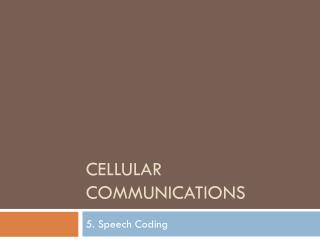Download PresentationCellular Communications

# Cellular Communications - PowerPoint PPT PresentationDownload Presentation## Cellular Communications

- - - - - - - - - - - - - - - - - - - - - - - - - - - E N D - - - - - - - - - - - - - - - - - - - - - - - - - - -
##### Presentation Transcript

1. Cellular Communications 5. Speech Coding

2. Low Bit-rate Voice Coding • Voice is an analogue signal • Needed to be transformed in a digital form (bits) • Speech signal is not random=>can be encoded using fewer bits as compared to random signal • If bits representing 1sec of speech can transferred over wireless channel during 200ms=> can pack 5 signals into the channel • For a handset transmitting less bits is alsoe means longer battery life

3. Requirement for speech coding • Can distort a speech a little bit (lossy) but should preserve acceptable quality • Shouldn’t be to complex • Use less power • Use less circuits • Reduce delay

4. Hierarchy of speech coders

5. Waveform Coders vs. VOCODERS • Waveform coders • Approximate any acoustic signal • VOCODERS • Based on prior knowledge of the signal • Speech signals are very special signals

6. Speech signals • Not all levels of a speech signal are equally likely • High probabilities of very low amplitudes • Significant probability of very high amplitudes • Monotonically decreasing probabilities of amplitudes between these two extremes • Speech is predictable • The next value of a speech signals can be predicted with large probability and fair precision from the past samples

7. Speech in frequency domain • Power of high frequency components is small • High frequency components when present are very important for speech quality

8. Sampling and quantization • Speech signal is analog, measured at infinitely many time instances and infinitely many possible values • Sampling: measure signal at finite time instances (sampling interval) • Quantization: approximate infinitely many possible values by finite number of possible values (e.g. 8 bits)

9. Uniform quantization • Divide the range of all possible values into finite number of equal intervals • Assign single quantization value to all values within the interval

10. Non-uniform quantization • Divide the range of all possible values into finite number of unequal but equally probable intervals • Logarithmic quantization: smaller intervals at low amplitudes • Different weight to low values • US: -Law • Europe: A-low

11. -Law

12. A-law

14. Rate-Distortion Theorem • Shannon: There existing a mapping from source waveform to code words such that for given distortion (error) D, R(D) bits per sample is sufficient to restore signal with an average distortion arbitrary close to D • R(D) is called rate distortion formula (achievable low bound) • Scalar quantization does not achieve this bound

15. Vector quantization • Encode a segment of sampled analog signal (e.g. L samples) • Use codebooks of n vectors • Segment all possible samples of dimension L into areas of equal probability • Very efficient at very low rates( R=0.5 bits per sample)

16. Learning codebook • LBG: Split areas (double codebook)

17. Adaptive Differential Pulse Code Modulation • PCM • Each sample representing by its amplitude (8 bits) • Standard telephony: 8K samples per second, 8 bit per sample= 64kbps • DPCM • Encode only difference from previous sample • Smaller differences are more often • Use less bits to represent smaller differences(4 bits) but more bits (10 bits) to represent larger differences

18. DPCM and prediction

19. ADPCM • Use more complex prediction in a transmitter/receiver to estimate next sample value • Transmitter send only difference between estimation and real value • Lossy codec: transmit approximate differences • Hopefully difference will be small

20. Frequency Domain Coding of Speech • Divide speech signal into a set of frequency components • Quantize and encode each component separately • Control number of bits/quality allocate to each band

21. Sub-band coding • Human ear does not detect error at all frequencies equally well

22. SBC

23. Vocoders • Model speech signal generation process • Transmitter analyze the voice signal according to assumed model • Transmitter sends parameters driveled from the analysis • Receiver synthesize voice based on received parameters • Vocoders are much more complex that waveform coders but achieve higher economy in a bit rate

24. Human Vocal Tract

25. Voice Generation Model

26. LPC

27. Advanced codecs • CELP • Transmitter/Receiver share common pitch codebook • Search for most suitable pitch code • RELP • Transmit model parameters • Also transmit Residual(differences) signal

28. Mean Opinion Score Quality Rating

29. Codec MOS rating